Impact Factor 2.323 | CiteScore 2.60
More on impact ›

# Frontiers in Computational Neuroscience## Original Research ARTICLE

Front. Comput. Neurosci., 14 July 2016 | https://doi.org/10.3389/fncom.2016.00064

# Visual Cortex Inspired CNN Model for Feature Construction in Text AnalysisHongping Fu1,Zhendong Niu1*,Chunxia Zhang2,Jing Ma1 andJie Chen1
• 1School of Computer Science and Technology, Beijing Institute of Technology, Beijing, China
• 2School of Software, Beijing Institute of Technology, Beijing, China

## 1. Introduction

Community Question Answering (CQA) has attracted a lot of attentions from both research and industry communities in recent years. A fundamental problem in CQA is answer recommendation, which recommends the best answer of a question to the asker who posts the question.

Most previous research takes this problem as a ranking task and employs learning-to-rank algorithms to rank answers. Then the answer in the top of the answer list is recommended to users. To achieve this, most researchers focus on constructing complex and novel features (e.g., lexical features, syntactic features, and semantic features) to improve the recommendation performance. For example, Surdeanu et al. (2011) use linguistically motivated features to rank answers to non-factoid questions. They exploit natural language processing such as named-entity identification, syntactic parsing, and semantic role labeling to construct similarity features, translation features, density features, and frequency features.

However, feature construction is a time-consuming and labor-consuming problem which needs huge priori knowledge and experience, especially with the increasingly huge amount of questions and corresponding answers in CQA. Nowadays, many researchers focus on constructing features automatically using neural network. They only focus on the information of questions and answers, which is not suitable for CQA containing huge social information. It is worth to leverage the social information presented in CQA that users tend to focus or vote answers according to the relation with others.

The rest of this paper is organized as follows. Section 2 describes the related work. The proposed BMFC-ARM is introduced in Section 3, which contains biological mechanism driven feature construction and answer ranking. Section 4 gives details of experiments and corresponding results. Finally, Section 5 summarizes conclusion and future work.

## 2. Related Work

### 2.2. Deep Learning for Text Analysis

Kalchbrenner et al. (2014) proposed a dynamic convolutional neural network with a dynamic k-Max pooling to model sentences. Hu et al. (2015) adapted the convolutional strategy in vision and speech, and then proposed convolutional neural network models for matching two sentences.

In classification tasks, Wang et al. (2016) proposed a framework to expand short texts based on word embedding clustering and convolutional neural network to overcome the worse classification performance caused by data sparsity and semantic sensitivity. Lai et al. (2015) introduce a recurrent neural network for text classification. Zeng et al. (2014) exploit convolutional neural network to extract word level features and sentence level features. Santos et al. (2015) proposed a a new pairwise ranking loss function and used convolutional neural network for relation classification.

Deep learning has been proven to be effective for many text analysis tasks. Recently some researchers brought deep learning into question answering. Bordes et al. (2014a,b) used an embedding model to project question-answer pairs into a joint space. tau Yih et al. (2014) used convolutional neural network to measure the similarity of entity and relation of a question with those in knowledge base for single-relation question answering. Iyyer et al. (2014) introduced a recursive neural network to model textual composition for factoid question answering. Zhou et al. (2016) aimed to find answers of previous queries to new queries, and used neural network architecture to learn the semantic representation of queries and answers in community question answering retrieval.

Different from previous deep learning methods only focusing on the semantics of question-answer pairs to rank answers, we also take user information into account in this paper, which is an very important aspect in community question answering.

## 3. Methodology

In this section, we present the proposed approach BMFC-ARM, which contains biological mechanism driven feature construction (BMFC) and answer ranking. First, an overview of the framework of BMFC-ARM is given. Then we describe the BMFC method and answer ranking in detail.

### 3.1. Overview of BMFC-ARM

Answer recommendation can be viewed as a ranking problem. Given a set of questions Q in a community question answering (CQA) system, each question qiQ contains a list of answers Ai = {ai1, ai2, …, aib, …, ain}, where aib is the best answer selected by asker or CQA systems, our goal is to learn a ranker according to these question-answer pairs, then recommend the best answer to any additional questions.

FIGURE 1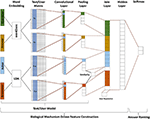Figure 1. The framework of BMFC-ARM, which contains two stages: BMFC and answer ranking. BMFC method is to automatically construct features by introducing the attention modulation and memory processing, which contains three parts: text model, user model, and feature fusion. The feature representation of questions, answers, and users are obtained from text model and user model respectively, and then the feature fusion constructs all feature representations together with the similarity of asker-answerer pairs and answerer's reputation into a combined feature vector. At last, Softmax is implemented to rank answers and recommend the best answer accordingly.

### 3.2. Biological Mechanism Driven Feature Construction (BMFC)

For the openness of CQA, all users can answer questions, which results in the unstable quality of answers. For the sociality of CQA, users get more interaction with each other when they are similar, and may select the answer that provided by the answerer who is similar with them as the best answer. Therefore, in this paper, we assume that when users choose an answer as the best answer in CQA, their thinking process have two properties: (1) whether the answer is related to the question; (2) whether the answerer is the person they care about or familiar with.

According to the assumption, we introduce attention modulation and memory processing of primate visual cortex, and propose a biological mechanism driven feature construction (BMFC) method. As users may choose an answer which answered by the person similar to them as the best answer, BMFC imitate the attention modulation property by computing the similarity between askers and answerers of given questions based on user model to reflect the relation between askers and answerers. The reputation information represents the quality of answers user answered. In order to reflect the relevance of answers and questions, BMFC method introduces user reputation to imitate the the memory processing property. BMFC method contains text model, user model and feature fusion. The flow of BMFC method is shown in Figure 2.

FIGURE 2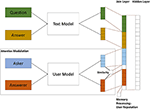Figure 2. The BMFC method, which contains three parts: text model, user model, and feature fusion. First, questions and their corresponding answers are passed through text model to get their feature vectors which contain semantic information. At the same time, the corresponding asker and answerer are passed through user model to get user feature vector. In order to introduce the attention modulation and memory processing properties, BMFC imitate the attention modulation property by introducing the asker-answerer information of given questions through user model and computing the similarity between them, and then bring in the user reputation information of user who answered the questions, which imitates the memory processing property. After getting the feature representation of questions, answers and users, feature fusion is used to combine those features into a single vector.

#### 3.2.1. Text Model

The text model in BMFC is based on convolutional neural network which is shown in Figure 3. It contains two channels to model question and answer respectively, and each channel contains a convolution layer followed by a simple pooling layer.

FIGURE 3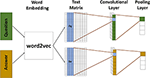Figure 3. The text model is used to map text into its corresponding feature representions. We use word2vec to tranform texts into vectors, and then use two channel convolutional neural network to model questions and answers. All texts pass through a convolutional layer followed by a pooling layer. After text model, texts are presented by their corresponding features.

##### 3.2.1.1. Text Matrix

Our text model first transforms the original text into vectors. Inspired by Kalchbrenner et al. (2014), we use word2vec that takes advantage of the context of the word which contains more semantic information to do the word embedding for each word in a text, and then construct the text matrix TRd × |t| shown bellow:

$T=[|||||w1⋯wi⋯w|t||||||]$

where ${\mathbf{w}}_{i}\in {\mathbf{R}}^{d}$ is the word embedding of a word in the text which contains |t| words and i is the position of the word in the text.

Then we will give a description of convolutional layer and pooling layer used in each channel in next sections.

##### 3.2.1.2. Convolutional layer

Convolutional layer is to convolve a matrix of weights with the matrix of activations at the layer below, which has two kinds of convolution: narrow convolution and wide convolution Kalchbrenner et al. (2014). In our framework, we use wide convolution which can deal with words at boundaries, and give equal attention to words in different positions. And we use ReLU as the activation function f(·). Given the text matrices TRd × |t| and a convolution filter kRm, the convolution operation between them results in a vector cR|t| + m −1. Each element of c is computed as follows:

where |t| is the number of text word, b is the bias, m is the width of convolutional filter.

##### 3.2.1.3. Pooling layer

After convolutional layer, the input texts are represented by the extracted features, and then passed through the pooling layer. Pooling layer is used to reduce the dimension of features obtained through the convolutional layer and aggregate feature information from different parts. There are three commonly used pooling methods: average-pooling, max-pooling, and stochastic-pooling. Boureau et al. (2010) compared average-pooling and max-pooling in detail. In this paper, we use max pooling which is the most widely used pooling methods. It chooses the feature with the maximum value in an area as shown in Equation (2).

Then, the text matrix, convolutional layer and pooling layer form our text model which builds rich feature representations of the input question and answer.

Unlike previous works which just map the question and answer into a vector space, BMFC takes user information into account modeling asker and answerer into the same vector space, and evaluates the relatedness of asker-answerer pairs based on user model.

#### 3.2.2. User Model

To introduce the attention modulation and memory processing property into BMFC, we propose user model which represents user information. In this paper, we use users' self descriptions as user information.

Same as text model, user model is based on CNN which contains two channels to represent askers and answerers, respectively. And each channel has a convolutional layer and a pooling layer shown in Figure 4. Since users' self descriptions are very short, e.g., some just contain keywords, we use Latent Dirichlet Allocation (LDA) Blei et al. (2003) to generate user matrix URd × |u|.

$U=[|||||wu1⋯wui⋯wu|u||||||]$

where d is the dimension of word vector, ${\mathbf{w}}_{ui}\in {\mathbf{R}}^{d}$ is the word representation of a word in user self description, |u| is the number of words and i is the position of wui.

FIGURE 4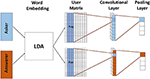Figure 4. The user model for mapping user information into its corresponding feature representation. We use LDA to generate user matrix, and then use two channel convolutional neural network to model askers and answerers. All user matrixes pass through a convolutional layer followed by a pooling layer. After user model, askers, and answerers are presented by their corresponding features.

The convolutional layer and pooling layer in user model are similar with those in text model.

#### 3.2.3. Feature Fusion

After text model and user model, the information of questions, answers, and corresponding askers and answerers is represented by numeric vectors vq, va, vuq, and vua, respectively. Then, we compute the similarity between asker and answerer to represent their relations. Here, we use cosine similarity shown as follows:

where suqua is the similarity between asker and answerer, ||υuq|| is the Euclidean norm of υuq = υuq1, υuq2, ⋯ , υuqn defined as $\sqrt{{\upsilon }_{uq1}^{2}+{\upsilon }_{uq2}^{2}+\cdots +{\upsilon }_{uqn}^{2}}$. Similarly, ||υua|| is the Euclidean norm of υua.

Then, BMFC method concatenates the asker-answerer similarities suqua, answerer's reputation vr, and corresponding vectors of question, answer, asker, and answerer into a single vector which can be represented as $\upsilon =\left[{{\upsilon }_{q}}^{T};{{\upsilon }_{a}}^{T};{{\upsilon }_{uq}}^{T};{s}_{uqua};{{\upsilon }_{ua}}^{T};{v}_{r}\right]$. Then, BMFC uses a hidden layer to interact the different parts of υ to construct the final feature to represent samples:

$ϕ(w·υ+b)$

where w is the weight vector of the hidden layer, b is the bias, and ϕ(·) is the tanh function.

### 3.3. Answer Ranking

After feature construction using BMFC method, question-answer pairs, and their corresponding users' information are represented through a vector V. In our method, we use a simple pointwise ranking method to rank answers. Softmax is often used in classification problem, which gives a probability of the sample belongs to each class. Given the sample vector V, the probability that it belongs to class j (j = 1, …, K) is computed by Equation (4). Then, answers are ranked according to this probability.

where Wk is the weight vector of the kth class.

## 4. Experiment

### 4.1. Experiment Setting

#### 4.1.1. Dataset

In our experiments, the raw data we use are from Stack Exchange Data Dump1, which is an anonymized dump of all user-contributed content on the Stack Exchange network2. The dataset contains 238 sites and each site consists of questions and corresponding answers of each question. We select around 840 resolved questions in four sites: movies, sports, travel, and music.

We split the dataset of 2385 question-answer pairs into a training set (train, 80%), a development set (dev, 10%), and a testing set (text, 10%) by randomly selecting 669 questions for training set, 87 questions for development set, and 84 questions for testing set, which are shown in Table 1. Here, each pair of question and its answer together with the corresponding asker and answerer constitutes an example. The example with best answer is considered as the most relevant example among all examples with other answers of the same question. This setup is used in training set, development set, and testing set.

TABLE 1

#### 4.1.2. Word Embeddings

In our experiments, we use word2vec3 to get word embeddings for questions and answers in text model, while using LDA to generate user representation for askers and answerers in user model.

For text model which represents question and answer information, we use word2vec to get word embeddings, which contains more semantic information by making use of the context of words. Similar with Kim (2014), Yu et al. (2014), we use the fixed word embeddings trained on all sites of Stack Exchange Data Dump. And we use the skipgram model with window size 5 to train word embeddings. Then words are represented by 50-dimensional vectors.

Due to the brief self description of users, we use JGibbLDA4 trained by Gibbs sampling to generate word embeddings for the user model. The parameter α is set as 0.5, β is set as 0.1, topic number is set as 100, and each topic contains 50 words.

#### 4.1.3. Parameters

The width mt of convolutional filter of the text model is set to 5, and the width mu of the user model is set to 2 according to experimental results. The convolutional maps of both models are 100, and the depth of the convolutional filter is set to 50 which is equal to the dimension of word vectors. We use ReLU as the activation function and max pooling method.

Similary with Kim (2014), we use stochastic gradient descent over mini-batches to train the BMFC-ARM where batch size is set to 50.

#### 4.1.4. Evaluation

For the task of answer recommendation, top answers in ranking list determine users' satisfaction. Therefore, we use Precision@N and Mean Reciprocal Rank(MRR) as metrics to evaluate our proposed method, which consider the position factor. Both of them are commonly used in information retrieval and question answering. Since we want to recommend the best answer to users, we use Precision@1(N = 1) in this paper, which means that we only focus on the precision of the first answer. Then Precision@1 is set to 1 if the best answer is ranked as first, 0 otherwise.

MRR takes the position of relevant answers into consideration. Where Reciprocal Rank is the multiplicative inverse of the rank of the first correct answer, and Mean Reciprocal Rank is the average of Reciprocal Rank that taken over all questions. MRR is computed as

$MRR=1|Q|∑q=1|Q|1rank(q)$

where |Q| is the number of questions in test dataset, rank(q) is the position of the best answer in the resulting answer list.

### 4.2. Results

When users' information is added, we compare the effects of different widths mu of convolution filter due to the brief self description of users. Unlike setting 5 as the width of convolution filter in the text model of representing questions and answers, our experiments compare the user model setting 2, 3, 4, and 5 as the width of convolution filter, respectively. Table 2 gives the answer recommending results using different convolution filter widths in the user model. As seen from Table 2, when mu = 2, the value of MRR and P@1 of all methods are higher than the cases of mu = 3, 4, and 5. The reason behind this result may be due to the brief user information. Therefore, in the subsequent experiments of this paper, the convolution filter width of the user model is set to 2.

TABLE 2

Figures 5, 6 show the recommendation results with different methods with memory processing property and without memory processing property. Figure 5 shows the results with MRR measure and Figure 6 gives the P@1 measure. In these two figures, the blue histogram represents methods which do not consider the memory process mechanism, while the red histogram represents methods that considered the memory process mechanism. From Figure 5 we can see that CNN-2, CNN-4, and CNN-4A obtain better performance by introducing the memory process mechanism, which shows that the memory processing mechanism through user reputation is useful to recommend best answers. For CNN-2 which just considers question information and answer information through two channels of CNN, the recommendation result performs better through adding user reputation, which shows that memory processing mechanism plays an important role in answer recommendation. From the recommendation results shown in Figure 6 we can find that P@1 measure has the same tendency with MRR measure, which also shows that methods with memory processing mechanism get better performance than those without memory processing mechanism.

FIGURE 5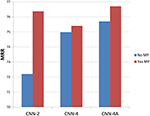Figure 5. Results with different methods along with memory property information (MRR). No MP means that methods do not introduce the memory property, where Yse MP means that methods considered the memory property.

FIGURE 6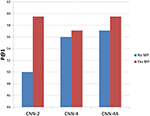Figure 6. Results with different methods along with memory property information (P@1). No MP means that methods do not introduce the memory property, where Yse MP means that methods considered the memory property.

The recommendation results of different methods (BMFC-ARM, CNN-1, CNN-2, CNN-4, CNN-4M, and CNN-4A) with evaluation metrics of MRR and P@1 are shown in Table 3. From Table 3, it is promising to observe that the proposed BMFC-ARM outperforms those CNN-1, CNN-2, CNN-4, CNN-4M, and CNN-4A with MRR and P@1 measure. It is probably because that BMFC-ARM takes user information into account introducing the attention modulation property and memory processing property. From methods CNN-2 and CNN-4, we can find that CNN-4 with user information performs better than CNN-2 which just uses question and answer information. This shows the importance of user information for answer recommendation. Therefore, when recommending the best answer to users in CQA, we need to take the relation information between askers and answerers into account rather than just considering question and answer information. The phenomenon that CNN-4M performs better than CNN-4 may be caused by the introduced memory processing property. This indicates that our memory processing property is useful by introducing user reputation information. And the phenomenon that CNN-4A performs better than CNN-4 shows that recommendation results can be improved by considering the similarity between askers and answerers which brings in users' relation. The method CNN-4A outperforms CNN-2 shows that through introducing the attention modulation property, represented by user information and the similarity between askers and answers, can improve the recommendation results.

TABLE 3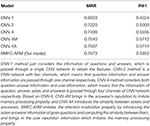Table 3. Results with different methods (CNN-1, CNN-2, CNN-4, CNN-4M, CNN-4A, and BMFC-ARM).

## 5. Conclusion

In the future, we will investigate how to bring the users' sentiment information of questions into our framework and find a novel way to represent the text.

## Author Contributions

HF prepared the methods of feature construction and answer ranking. JC provided the related researches. HF and JM conducted the experiments. HF prepared the manuscript. ZN and CZ initiated this study and supervised all aspects of the work. All authors discussed the results and commented on the manuscript.

## Conflict of Interest Statement

The authors declare that the research was conducted in the absence of any commercial or financial relationships that could be construed as a potential conflict of interest.

## Acknowledgments

The authors wish to acknowledge the support of the National 973 Project of China (No. 2012CB720702), the National Natural Science Foundation of China (Project No. 61370137, No. 61272361) and Major Science and Technology Project of Press and Publication (No: GAP- P ZDKJ BQ/01).

## References

Blei, D. M., Ng, A. Y., and Jordan, M. I. (2003). Latent dirichlet allocation. J. Mach. Learn. Res. 3, 993–1022. doi: 10.1162/jmlr.2003.3.4-5.993

Bordes, A., Chopra, S., and Weston, J. (2014a). “Question answering with subgraph embeddings,” in Proceedings of the 2014 Conference on Empirical Methods in Natural Language Processing, EMNLP (Doha), 615–620.

Bordes, A., Weston, J., and Usunier, N. (2014b). “Open question answering with weakly supervised embedding models,” in Proceedings of the European Conference on Machine Learning and Knowledge Discovery in Databases - Vol. 8724, ECML PKDD 2014, (New York, NY: Springer-Verlag New York, Inc), 165–180.

Boureau, Y. L., Bach, F., LeCun, Y., and Ponce, J. (2010). “Learning mid-level features for recognition,” in 2010 IEEE Conference on Computer Vision and Pattern Recognition, CVPR (San Francisco, CA), 2559–2566.

Dalip, D. H., Gonçalves, M. A., Cristo, M., and Calado, P. (2013). “Exploiting user feedback to learn to rank answers in q&a forums: a case study with stack overflow,” in Proceedings of the 36th International ACM SIGIR Conference on Research and Development in Information Retrieval, SIGIR '13, (New York, NY: ACM), 543–552.

Hieber, F., and Riezler, S. (2011). “Improved answer ranking in social question-answering portals,” in Proceedings of the 3rd International Workshop on Search and Mining User-generated Contents, SMUC '11, (New York, NY: ACM), 19–26.

Hu, B., Lu, Z., Hang, L., and Chen, Q. (2015). “Convolutional neural network architectures for matching natural language sentences” in Advances in Neural Information Processing Systems 27 – 28th Annual Conference on Neural Information Processing Systems 2014, NIPS 2014, (Montreal, QC).

Iyyer, M., Boyd-Graber, J., Claudino, L., Socher, R., and Iii, H. D. (2014). “A neural network for factoid question answering over paragraphs,” in Proceedings of the 2014 Conference on Empirical Methods in Natural Language Processing, EMNLP (Doha), 633–644.

Kalchbrenner, N., Grefenstette, E., and Blunsom, P. (2014). “A convolutional neural network for modelling sentences,” in Proceedings of the 52nd Annual Meeting of the Association for Computational Linguistics (Baltimore, MD), 655–665.

Kim, Y. (2014). “Convolutional neural networks for sentence classification,” in Proceedings of the 2014 Conference on Empirical Methods in Natural Language Processing, EMNLP (Doha), 1746–1751.

Lai, S., Xu, L., Liu, K., and Zhao, J. (2015). “Recurrent convolutional neural networks for text classification,” in Proceedings of the Twenty-Ninth AAAI Conference on Artificial Intelligence, AAAI, 15 (Austin, TX), 2267–2273.

Liu, X., Xie, R., Lin, C., and Cao, L. (2014). Question microblog identification and answer recommendation. Multimedia Syst. 22, 487–496. doi: 10.1007/s00530-014-0411-z

Santos, C. N. D., Xiang, B., and Zhou, B. (2015). “Classifying relations by ranking with convolutional neural networks,” in Proceedings of the 53rd Annual Meeting of the Association for Computational Linguistics and the 7th International Joint Conference on Natural Language Processing (Beijing), 626–634.

Surdeanu, M., Ciaramita, M., and Zaragoza, H. (2011). Learning to rank answers to non-factoid questions from web collections. Comput. Linguist. 37, 351–383. doi: 10.1162/COLI_a_00051

tau Yih, W., He, X., and Meek, C. (2014). “Semantic parsing for single-relation question answering,” in Proceedings of the 52nd Annual Meeting of the Association for Computational Linguistics (Baltimore, MD), 643–648.

Tu, X., Wang, X.-J., Feng, D., and Zhang, L. (2009). “Ranking community answers via analogical reasoning,”in Proceedings of the 18th International Conference on World Wide Web, WWW '09, (New York, NY: ACM), 1227–1228.

Wang, P., Xu, B., Xu, J., Tian, G., Liu, C.-L., and Hao, H. (2016). Semantic expansion using word embedding clustering and convolutional neural network for improving short text classification. Neurocomputing 174, 806–814. doi: 10.1016/j.neucom.2015.09.096

Wang, X.-J., Tu, X., Feng, D., and Zhang, L. (2009). “Ranking community answers by modeling question-answer relationships via analogical reasoning,” in Proceedings of the 32Nd International ACM SIGIR Conference on Research and Development in Information Retrieval, SIGIR '09, (New York, NY: ACM), 179–186.

Yu, L., Hermann, K. M., Blunsom, P., and Pulman, S. (2014). “Deep learning for answer sentence selection” in Proceedings of the Deep Learning and Representation Learning Workshop: NIPS-2014 (Montreal, QC).

Zeng, D., Liu, K., Lai, S., Zhou, G., and Zhao, J. (2014). “Relation classification via convolutional deep neural network,” in the 25th International Conference on Computational Linguistics: Technical Papers, COLING 2014 (Dublin), 2335–2344.

Zhou, G., Zhou, Y., He, T., and Wu, W. (2016). Learning semantic representation with neural networks for community question answering retrieval. Knowl. Based Syst. 93, 75–83. doi: 10.1016/j.knosys.2015.11.002

Zhou, Z.-M., Lan, M., Niu, Z.-Y., and Lu, Y. (2012). “Exploiting user profile information for answer ranking in cqa,” in Proceedings of the 21st International Conference Companion on World Wide Web, WWW '12 Companion (New York, NY: ACM), 767–774.

Keywords: convolutional neural networks, biologically inspired feature construction, feature encoding, answer recommendation, community question answering, text analysis

Citation: Fu H, Niu Z, Zhang C, Ma J and Chen J (2016) Visual Cortex Inspired CNN Model for Feature Construction in Text Analysis. Front. Comput. Neurosci. 10:64. doi: 10.3389/fncom.2016.00064

Received: 31 March 2016; Accepted: 13 June 2016;
Published: 14 July 2016.

Edited by:

Hong Qiao, Institute of Automation, China

Reviewed by:

Junfa Liu, Institute of Computing Technology, China
Feng Tian, Institute of Software, China

Copyright © 2016 Fu, Niu, Zhang, Ma and Chen. This is an open-access article distributed under the terms of the Creative Commons Attribution License (CC BY). The use, distribution or reproduction in other forums is permitted, provided the original author(s) or licensor are credited and that the original publication in this journal is cited, in accordance with accepted academic practice. No use, distribution or reproduction is permitted which does not comply with these terms.

*Correspondence: Zhendong Niu, zniu@bit.edu.cn